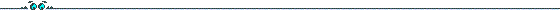# Abstract for talk at YACC 2000#### Trace-Zero Subvariety for Cryptosystems

We present a kind of groups suitable for cryptographic applications: the trace-zero subvariety. The construction is based on Weil descent from curves of genus one or two over extension fields \$\F_{p^n}\$, \$n=3\$ (or 5).
On the Jacobian of the curve the group can be seen as a prime order subgroup, however, considering the construction as Weil descent reveals that the security is equivalent to that of groups based on low-genus hyperelliptic curves over prime fields.
The advantage is that the complexity to compute scalar multiples is lower in most cases as one can make use of the Frobenius endomorphism of the initial curve.
Thus the trace-zero subvariety can be used efficiently in protocols based on the discrete logarithm problem.

back
zurück